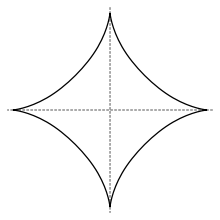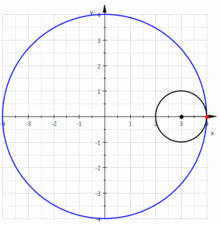# Astroid

Not to be confused with Asteroid.AstroidThe hypocycloid construction of the astroid.

An astroid is a particular mathematical curve: a hypocycloid with four cusps. Specifically, it is the locus of a point on a circle as it rolls inside a fixed circle with four times the radius. By double generation, it is also the locus of a point on a circle as it rolls inside a fixed circle with 4/3 times the radius. It can also be defined as the envelope of a line segment with an end point on each of the axes. It is therefore the envelope of the moving bar in the Trammel of Archimedes.

Its modern name comes from the Greek word for "star". The curve had a variety of names, including tetracuspid (still used), cubocycloid, and paracycle. It is nearly identical in form to the evolute of an ellipse.

## Equations

If the radius of the fixed circle is a then the equation is given byThis implies that an astroid is also a superellipse.The pedal equation with respect to the origin isthe Whewell equation isand the Cesàro equation isThe polar equation isThe astroid is a real locus of a plane algebraic curve of genus zero. It has the equationThe astroid is therefore a real algebraic curve of degree six.

## Metric properties

Area enclosedLength of curveVolume of the surface of revolution of the enclose area about the x-axis.Area of surface of revolution about the x-axis## Properties

The astroid has four cusp singularities in the real plane, the points on the star. It has two more complex cusp singularities at infinity, and four complex double points, for a total of ten singularities.

The dual curve to the astroid is the cruciform curve with equationThe evolute of an astroid is an astroid twice as large.

## References

1. Yates
2. Yates, for section
3. Mathworld
4. Yates, for section
• J. Dennis Lawrence (1972). A catalog of special plane curves. Dover Publications. pp. 4–5,34–35,173–174. ISBN 0-486-60288-5.
• Wells D (1991). The Penguin Dictionary of Curious and Interesting Geometry. New York: Penguin Books. pp. 1011. ISBN 0-14-011813-6.
• R.C. Yates (1952). "Astroid". A Handbook on Curves and Their Properties. Ann Arbor, MI: J. W. Edwards. pp. 1 ff.Wikimedia Commons has media related to Astroid.